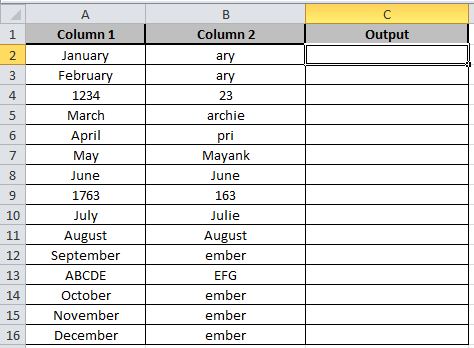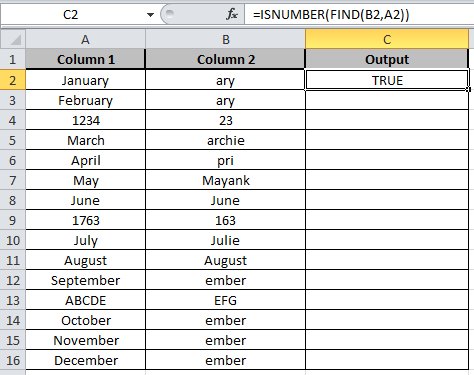# Searching a String for a Specific Substring in Excel

In this article, we will learn how to search a substring from a given string in Excel.

In excel, substring is a part of another string. It can be a single character or a whole paragraph. To search a string for a specific substring, we will use the ISNUMBER function along with the FIND function in Excel. Instead of FIND, you can always use the excel SEARCH function for non-case sensitive searches.

ISNUMBER function is used to check the cell if it contains a number or not.

Syntax of ISNUMBER:

 =ISNUMBER (value)

The FIND function returns the position of the character in a text string, reading left to right (case-sensitive).

Syntax of Find:

 =FIND(find_text,within_text,[start_num])Here we have two columns. Substring in Column B and Given string in Column A.

Write the formula in C2 cell

Formula:

 =ISNUMBER(FIND(B2,A2))

Explanation:

Find function takes the substring from the B2 cell of Column B and it then matches it with the given string in the A2 cell of Column A.

ISNUMBER checks if the string matches, it returns True else it returns False.Copy the formula in other cells, select the cells taking the first cell where the formula is already applied, use shortcut key Ctrl+ D.

As you can see the output in column C shows True and False representing whether substring is there or not.

Now, what if you want to do a non-case sensitive search in excel?

## How to search in excel cells for non-case sensitive substrings?

It's simple. Replace the find function with the excel SEARCH function in the formula above.  Since SEARCH is case-insensitive.

There are more articles on FIND and SEARCH function to find strings or value. Hope you learned how to find substring in a string from the article. You can perform these tasks in Excel 2016, 2013 and 2010. If you have any unresolved query, please do comment below in the comment box. We will help you.

Related Articles:

How to Search a certain text in Excel

How to Sum if cells contain specific text in Excel

How to Sum if cell contains text in other cell in Excel

How to Count Cells that contain specific text in Excel

How to Split Numbers and Text from String in Excel

How to Highlight cells that contain specific text in Excel

Popular Articles:

50 Excel Shortcut to Increase Your Productivity

How to use the VLOOKUP Function in Excel

How to use the COUNTIF function in Excel 2016

How to use the SUMIF Function in Excel

Terms and Conditions of use

The applications/code on this site are distributed as is and without warranties or liability. In no event shall the owner of the copyrights, or the authors of the applications/code be liable for any loss of profit, any problems or any damage resulting from the use or evaluation of the applications/code.